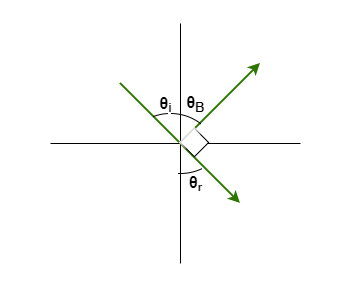# Problem: Light traveling in water of refractive index 1.33 is incident on a plate of glass of refractive index 1.53. At what angle of incidence will the reflected light be fully polarized?

###### FREE Expert Solution

Let's consider the diagram:From Brewster's angle law, we have:

θi = θB, where θi is the angle of incidence in the case where light is completely polarized.

93% (164 ratings)###### Problem Details

Light traveling in water of refractive index 1.33 is incident on a plate of glass of refractive index 1.53. At what angle of incidence will the reflected light be fully polarized?